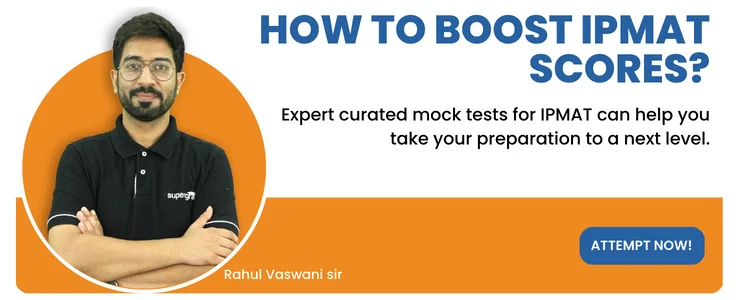• Home
• Number Theory Questi...

# Number Theory Questions for IPMAT Preparation

Author : Palak Khanna

Updated On : March 4, 2023

SHARE

Number theory is the field of mathematics associated with studying the properties and identities of integers. Most of you might feel that this topic is vast and difficult to study. However, you can score good marks on this topic if you are aware of the concepts.

Are you an aspirant for the IPM but don't know how to solve number theory questions? Well, you are in the right place!

This post takes you through the sample Number Theory Questions for IPMAT, previous year's questions, and more.

So, what are you waiting for? Practice these questions and enhance your preparation for the upcoming exam.

### Important Topics under Number Theory

There are numerous topics under the number theory concept. However, the following are some of the important topics under the syllabus for IPMAT.

• Divisibility
• Fractions
• LCM and HCM
• Prime Numbers
• Modular arithmetic
• Arithmetic functions
• Analytical number theory
• Algebraic number theory
• L-functions

## Sample Number Theory Questions for IPMAT

Practice is the key to perform well in the exam. Ensure that your basics are clear and have a good grip. You can pen down the topics in which you are weak and strong separately. This can help you to decide on which topic you need to focus more on.

You are advised to practice at least 1-2 previous year question papers for IPMAT on a weekly basis as this will help you to know the difficulty level and the type of questions asked in the exam.

Q) A number N=0.abcabcabc....where a,b,c are not simultaneously 0. By which of the following number N should be multiplied to get an integer product?

a) 99

b) 09

c) 3996

d) Noen of the above

Q) The total number of positive integers 'n' from 10 to 70 such that the product (n-1). (n-2)......3.2.1 is not divisible by 'n' is

a) 14

b) 15

c) 16

d) 17

Q) Let E be the set of even integers 'a' from 125 to 275 where 'a' is divisible by 9 but not by 11. How many elements does 'E' contain?

a) 12

b) 11

c) 8

d) 9

Q) If 'Z' is the LCM of the first 25 natural numbers, then find the LCM of the first 30 natural numbers.

a) 111 Z

b) 21X22X23X24X25 Z

c) 87 Z

d) 123 Z

Q) A number, when divided by 4, 5, and 7, successively leaves the remainders 2, 3, and 4, respectively. Find the remainder when the same number is divided by 70.

a) 20

b) 64

c) 24

d) 35

Q) A certain number K has 8 factors. Which among the following cannot be the possible number of factors of K^3?

a) 22

b) 27

c) 40

d) 64

Q) 48 students have to be seated such that each row has the same number of students as the others. If at least 3 students are to be seated per row and at least 2 rows have to be there, how many arrangements are possible?

a) 4

b) 10

c) 8

d) 7

Q) How many divisors does 7200 have?

a) 20

b) 4

c) 54

d) 32

Q) What is the value of M and N respectively? If M39048458N is divisible by 8 & 11; where M & N take integer values from 0 to 9, inclusive?

a) M = 7; N = 8

b) M = 8; N = 6

c) M = 6; N = 4

d) M = 5; N = 4

Q) What is the remainder when 11^29 is divided by 1332?

a) 121

b) 1331

c) 1211

d) 1### Previous Year Number Theory Questions for IPMAT

Solving previous year's questions is one of the best ways to enhance your preparation. Try to solve as many previous year question papers as possible to improve your speed.

To ease out your preparation, we have provided a few number theory questions that were asked in the previous year's IPMAT exam.

Q) What is the remainder when 5^83 is divided by 15?

a) 1

b) 5

c) 3

d) 0

Q) For what smallest natural number 'n', remainder by 651^n and 652^n when divided by 13 are equal?

a) 4

b) 6

c) 12

d) 15

Q) What is the remainder when 11^29 is divided by 1332?

a) 121

b) 1331

c) 1211

d) 1

Q) What is the remainder when 358^76 is divided by 14?

a) 4

b) 2

c) 8

d) 6

Q) What is the remainder when 65^19-42^19+66^19-43^19 divided by 46?

a) 45

b) 1

c) 0

d) 44

Q) N is an integer. If (N-5) is a multiple of 13, then the largest number that will always divide (N+8)(N+21) is

a) 13

b) 322

c) 169

d) 338

Q) Find the last two digits of the number N=199^43?

a) 09

b) 99

c) 89

d) 19

Read Also - IPMAT Verbal Ability Tips 2023

How can I prepare for the Number Theory Questions for IPMAT?How can I improve my accuracy while solving number theory questions in the IPMAT exam?Which is the best Study Material for IPMAT Quant Preparation?